# 13,12 M 7el V-Maco 12,31 dBI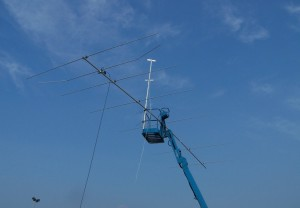For: DXing  AND Super Bowl !
Antenna Type:    7 elements V-Yagi
Designed by:    HPSD version 1.01 Mrch 2014
Boom length:     13,15 Meter
Gain:12,,31 dBI  (@27,555 MHz, )
FB            <29 dB
FR            <25 dB (az)
Impedance: 50 ohms, direct fed
SWR 1:1 500 KHz.
SWR below 2:1    > 1300 KHz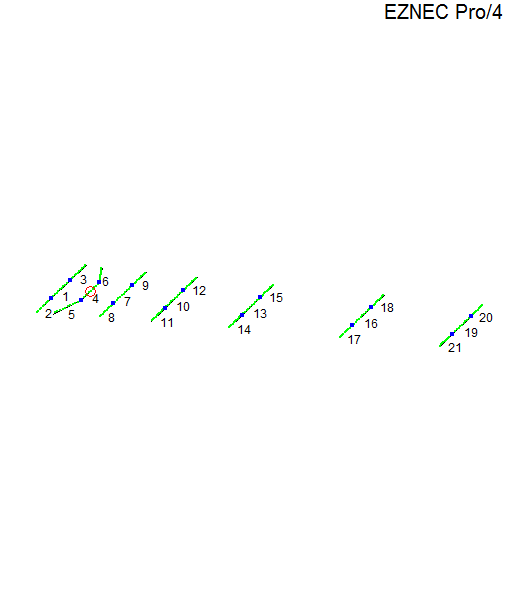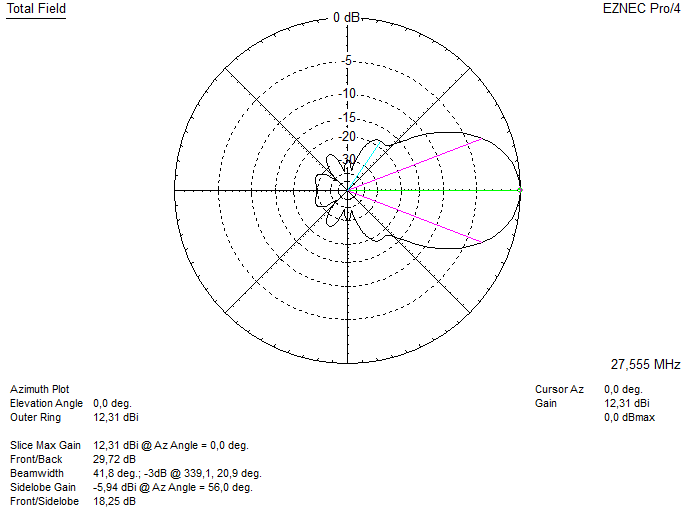Above: The freespace azimuth plot. (including aluminium loss).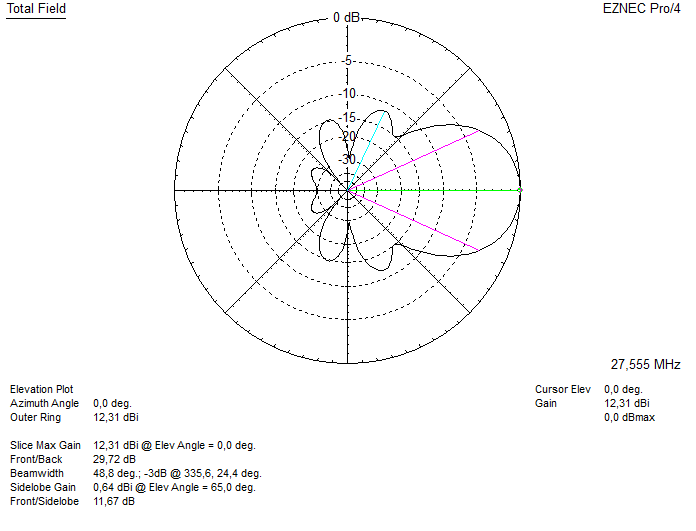Above: The freespace elevation plot !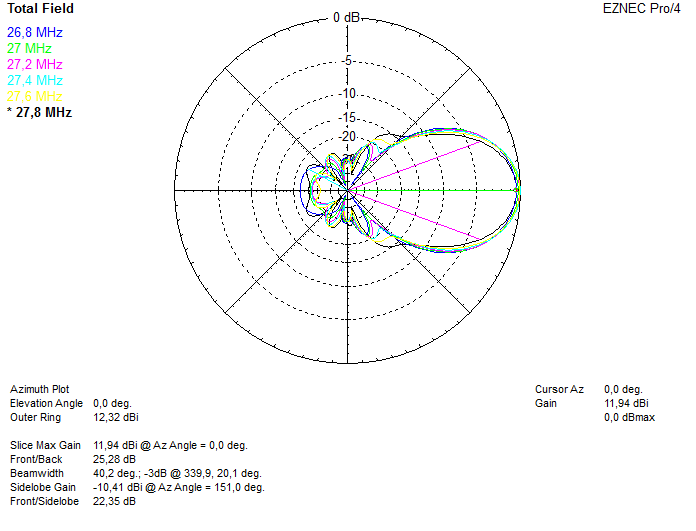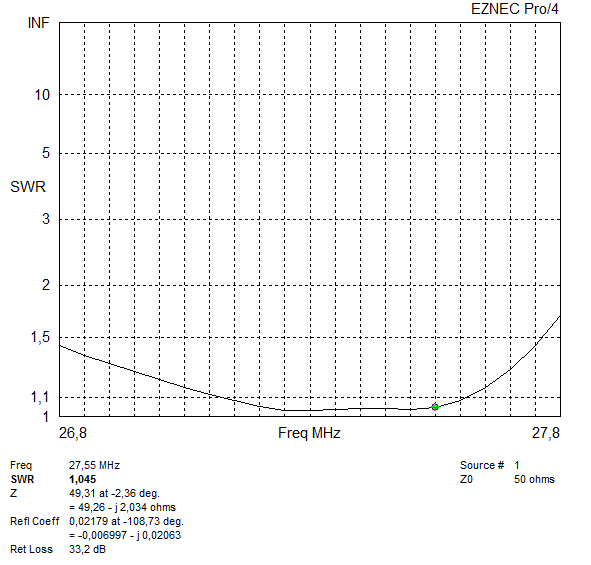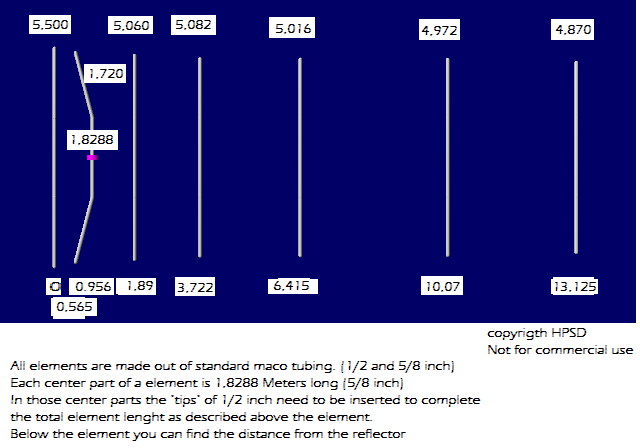• The 5/8inch 1,8288 meter long part is straight.
• The bend starts there where the 1/2 inch tubing starts.
• At that point the radiator is 0,956 Meter seperated from the reflector
• At the end of the radiator the radiator is 0,565 Meter seperated from the reflector
• In total the length of the 1/2 inch part should be 1,720 Meters ,making the total lenght of the radiator: 5,2688 Meter.

DO NOT CHANGE DIAMETERS.

any questions ? please use the forum. (top)

Not for commercial use.

VERSION DWAIN: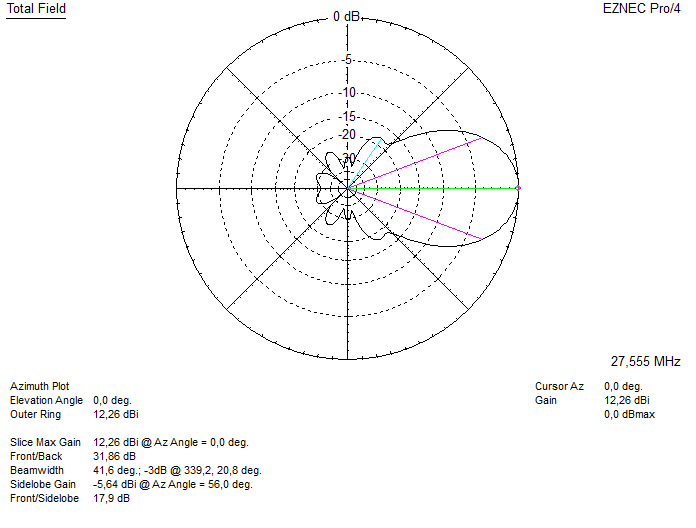Above the freespace azimuth plot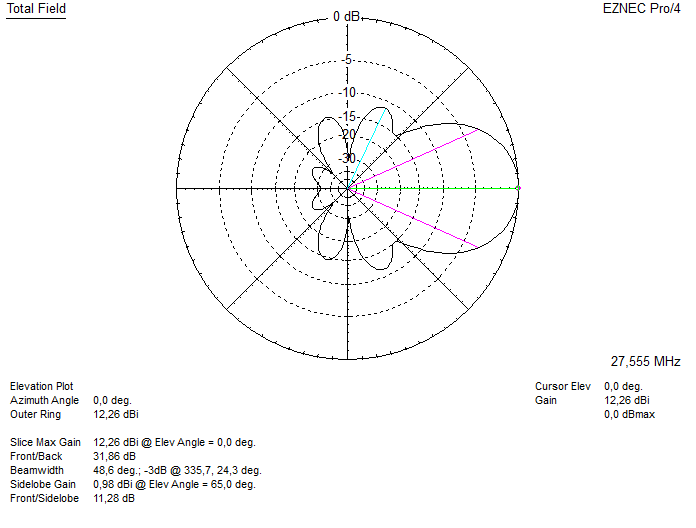Above the freespace elevation plot.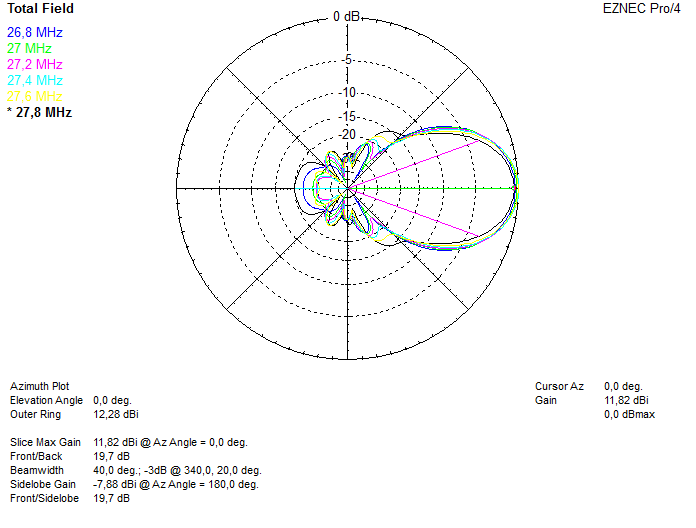Above the freespace azimuth plot at different frequencies.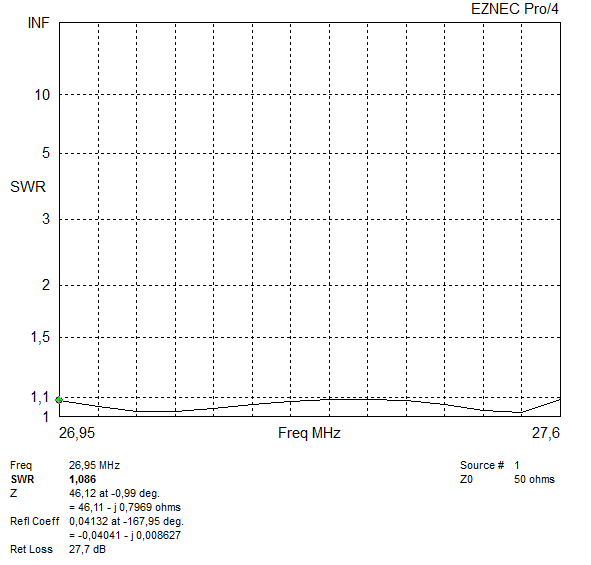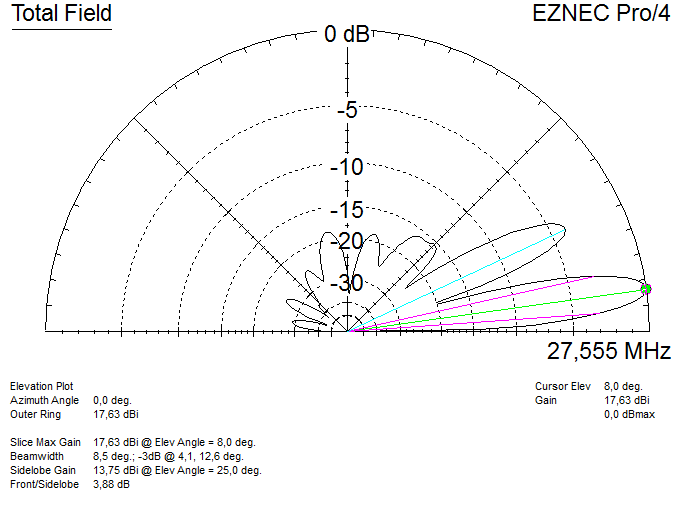Above the elevation plot when placed at 18 meters above average ground.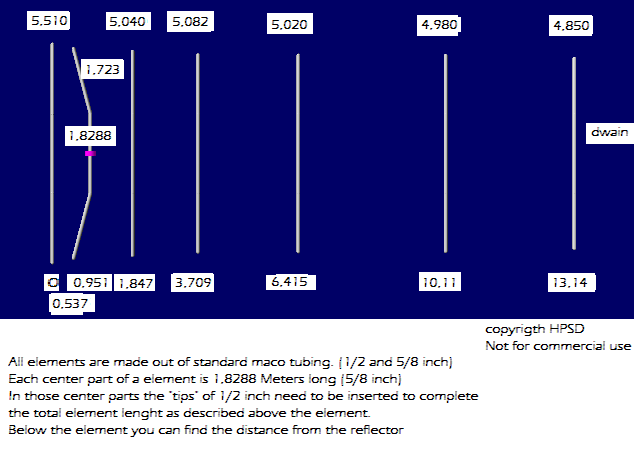• The 5/8inch 1,8288 meter long part is straight.
• The bend starts there where the 1/2 inch tubing starts.
• At that point the radiator is 0,956 Meter seperated from the reflector
• At the end of the radiator the radiator is 0,565 Meter seperated from the reflector
• In total the length of the 1/2 inch part should be 1,720 Meters ,making the total lenght of the radiator: 5,2688 Meter.

DO NOT CHANGE DIAMETERS.

any questions ? please use the forum. (top)